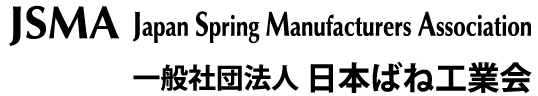# Heating Element Design and Calculation

To perform as a heating element the tape or wire must resist the flow of electricity. This resistance converts the electrical energy into heat which is related to the electrical resistivity of the metal, and is defined as the resistance of a unit length of unit cross-sectional area. The linear resistance of a length of tape or wire may be calculated from its electrical resistivity.

Where:

#### For Tape

As a heating element, tape offers a large surface area and therefore, a greater effective heat radiation in a preferred direction making it ideal for many industrial applications such as injection mould band heaters.

An important characteristic of these electrical resistance alloys is their resistance to heat and corrosion, which is due to the formation of oxide surface layers that retard further reaction with the oxygen in air. When selecting the alloy operating temperature, the material and atmosphere with which it comes into contact must be considered. As there are so many types of applications, variables in element design and different operating conditions the following equations for element design are given as a guide only.

#### Electrical Resistance at Operating Temperature

With very few exceptions the resistance of a metal will change with temperature, which must be allowed for when designing an element. As the resistance of an element is calculated at operating temperature, the resistance of the element at room temperature must be found. To obtain the elements resistance at room temperature, divide the resistance at operating temperature by the temperature resistance factor shown below:

Where :

F = Temperature-Resistance Factor
Rt = Element resistance at operating temperature (Ohms)
R = Element resistance at 20°C (Ohms)

RW45 changes little in resistance as temperature rises, having a temperature-resistance factor of +0.00003/°C in the 20 – 100°C range.